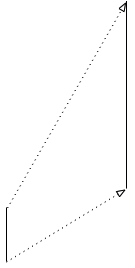# bjorg

## Saturday, September 21, 2013

### Mapping ParametersVisualizing a Linear Mapping
Very often we need to "map" one set of values to another. For example, if we have a slider that ranges from 0 to 1, and we want to use it to control the value of a frequency setting. Or perhaps we have the output of a sine wave (which ranges from -1 to 1) and we want to use that to control the intensity of a EQ. In these cases and many more, we can use a linear mapping to get from one range of values to another.

A linear mapping is simply a linear equation, such as y = mx + b, that takes an input, your slider value for example, and gives you back an output. The input is x, and the output is y. The trick is to find the values of m and b.

Let's take a concrete example. Let's say you have the output of a sine wave (say from an LFO) that oscillates between -1 and 1. Now we want to use those values to control a frequency setting from 200 to 2000. In this case, x from the equation above represents the oscillator, and y represents the frequency setting.

We know two things: we want x=-1 to map to y=200, and x=1 to map to y=2000. Since our original equation, y = mx + b, had two unknowns (m and b), we can solve it:

Original equation with both unknowns:
y = mx + b

Substituting our known values for x and y:
200 = (-1)m + b
2000 = (1)m + b

Solving for b:
2200 = 2b
1100 = b

Solving for m:
2000 = m + 1100
900 = m

Final equation:
y = 900x + 1100

You can check the final equation by substituting -1 and 1 for x and making sure you get 200 and 2000 respectively for y.

So in our LFO/frequency example, we would take our LFO value, say .75, and use that as x. Then plug that value into the formula (y=900(.75) + 1100=1775) and get our final value for our frequency setting.